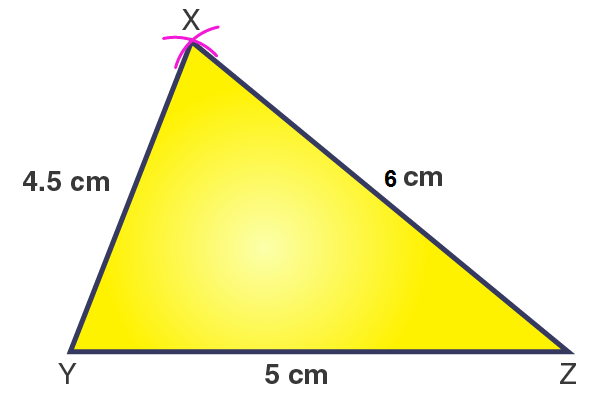# Construct ΔXYZ in which XY = 4.5 cm, YZ = 5 cm and ZX = 6 cm

SolutionThe steps for construction are given below:

1. Draw a line segment YZ = 5 cm.

2. Considering Z as a center and radius 6 cm, draw an arc.

3. Considering Y as a center and radius 4.5 cm, draw another arc, cutting the previous arc at X.

4. Join XY and XZ.

Hence, ΔXYZ obtained is the required triangle.(0)(0)